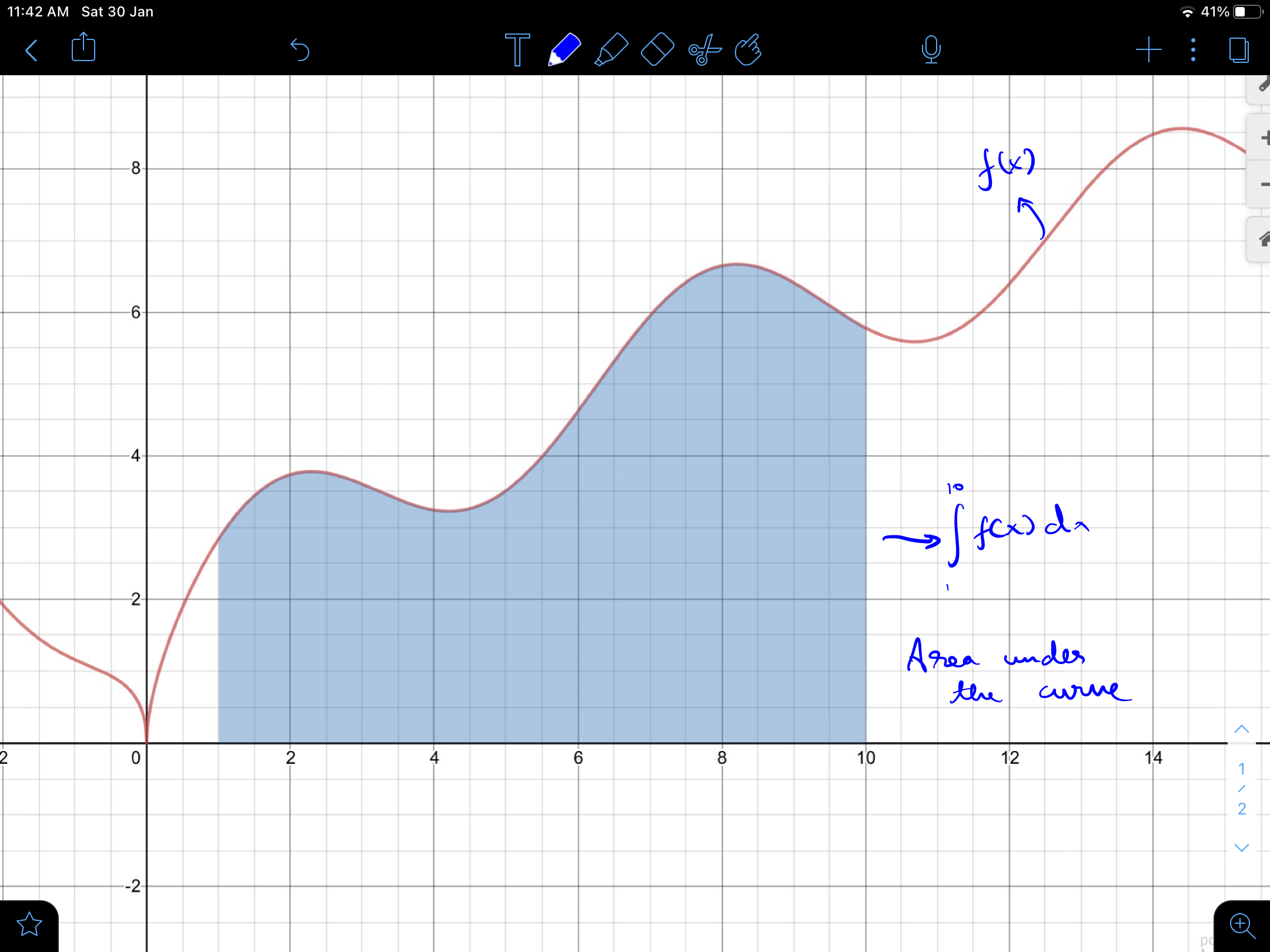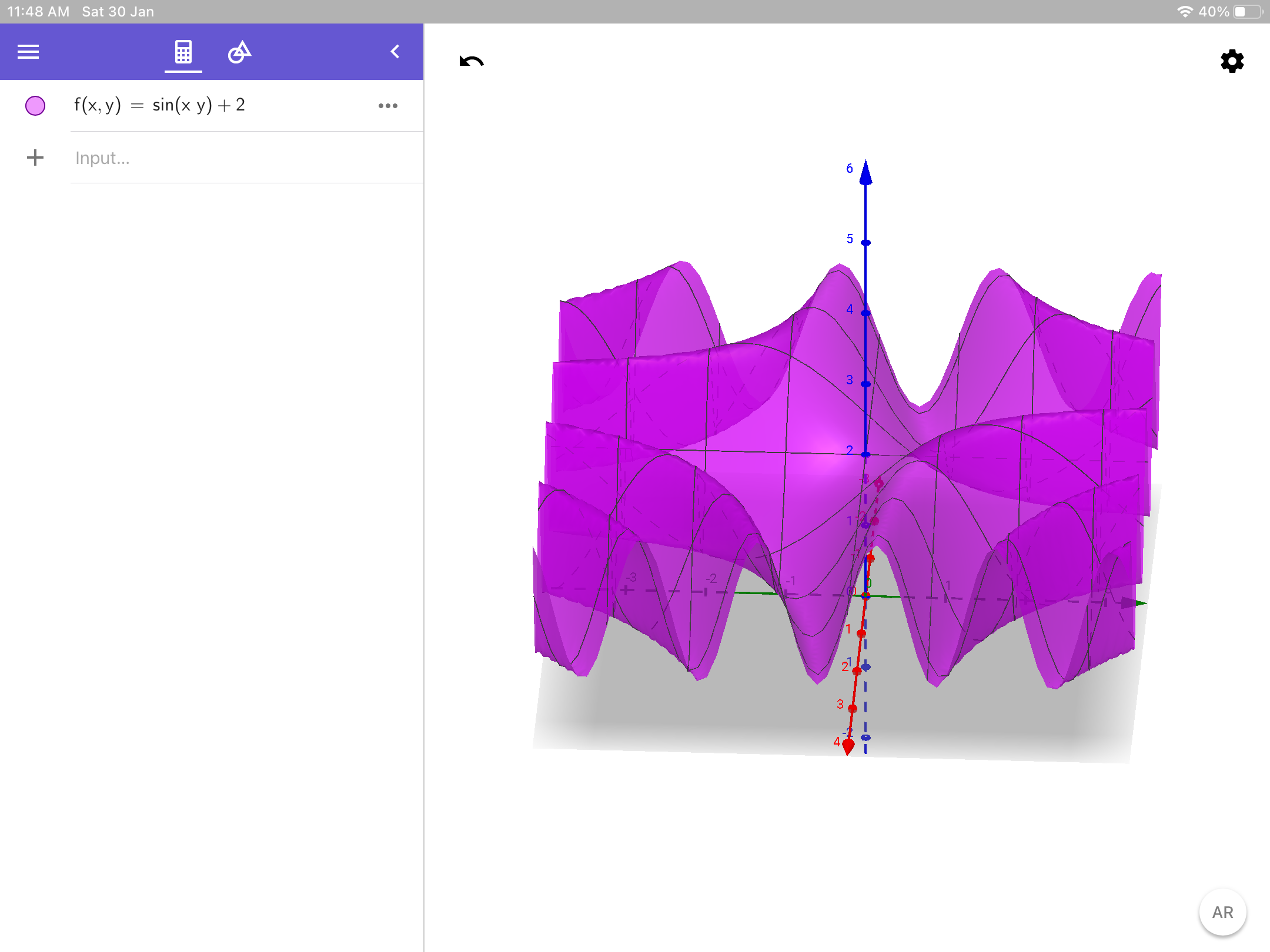# Question involving the double integral

From this wiki page multiple integral, the multiple integral is "an integral of a function over a two-dimensional region". But the caption of the image below states that "The double integral of the graphed function corresponds to the volume contained underneath the surface corresponding to the function." and volume is three-dimensional. What is this? Why is it contradicting? Am I missing something?Note by Harry Lam
6 months ago

This discussion board is a place to discuss our Daily Challenges and the math and science related to those challenges. Explanations are more than just a solution — they should explain the steps and thinking strategies that you used to obtain the solution. Comments should further the discussion of math and science.

When posting on Brilliant:

• Use the emojis to react to an explanation, whether you're congratulating a job well done , or just really confused .
• Ask specific questions about the challenge or the steps in somebody's explanation. Well-posed questions can add a lot to the discussion, but posting "I don't understand!" doesn't help anyone.
• Try to contribute something new to the discussion, whether it is an extension, generalization or other idea related to the challenge.

MarkdownAppears as
*italics* or _italics_ italics
**bold** or __bold__ bold
- bulleted- list
• bulleted
• list
1. numbered2. list
1. numbered
2. list
Note: you must add a full line of space before and after lists for them to show up correctly
paragraph 1paragraph 2

paragraph 1

paragraph 2

[example link](https://brilliant.org)example link
> This is a quote
This is a quote
    # I indented these lines
# 4 spaces, and now they show
# up as a code block.

print "hello world"
# I indented these lines
# 4 spaces, and now they show
# up as a code block.

print "hello world"
MathAppears as
Remember to wrap math in $$ ... $$ or $ ... $ to ensure proper formatting.
2 \times 3 $2 \times 3$
2^{34} $2^{34}$
a_{i-1} $a_{i-1}$
\frac{2}{3} $\frac{2}{3}$
\sqrt{2} $\sqrt{2}$
\sum_{i=1}^3 $\sum_{i=1}^3$
\sin \theta $\sin \theta$
\boxed{123} $\boxed{123}$

Sort by:

Well a single integral is the area under the curve so add a dimension to both

- 6 months ago

Add a dimension to both what? The x and y axis? Now there are three dimensions - for finding the volume. But the definition says "the multiple integral is an integral of a function over a two-dimensional region" which contradicts this

- 6 months ago

Sorry I had meant add a dimension to the function, and add a dimension to the integral

The function was a curve previously, or 1 dimensional but now is 2d wavy sheet taking inputs from x and y and outputting as z

The single integral was the area under a 1 dimensional curve and now becomes the volume under a 2 dimensional wavy sheetThe volume under this is the double integral depending on what limits are taken

- 6 months ago

Ok I see. But couldn't you do the same thing by making a solid of revolution to find the volume? Is that different?

- 6 months ago

I think that’s only possible when the figure has circular symmetry to exploit otherwise a change to the cylindrical co-ordinate system needs to be done ( I think that this is very hard to perform especially on random functions ) and also the limits will have to be well placed

I am very novice to double integrals, so I have very limited knowledge on them

- 6 months ago

OK I see now. Thanks @Jasan Gomez for explaining it to me!

- 6 months ago

Solids of revolutions have one less degree of freedom than usual so they can be solved using single integrals itself ( but these single integrals are integrating areas into volumes, normally it integrates line segments into areas, look up on riemann sums if you didn’t understand the integrating line segments into area part )

- 6 months ago